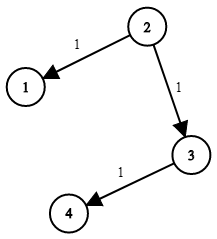# 743. Network Delay Time | LeetCode Solution

## 743. Network Delay Time | LeetCode Solution

You are given a network of `n` nodes, labeled from `1` to `n`. You are also given `times`, a list of travel times as directed edges `times[i] = (ui, vi, wi)`, where `ui` is the source node, `vi` is the target node, and `wi` is the time it takes for a signal to travel from source to target.

We will send a signal from a given node `k`. Return the time it takes for all the `n` nodes to receive the signal. If it is impossible for all the `n` nodes to receive the signal, return `-1`.

Example 1:```Input: times = [[2,1,1],[2,3,1],[3,4,1]], n = 4, k = 2
Output: 2
```

Example 2:

```Input: times = [[1,2,1]], n = 2, k = 1
Output: 1
```

Example 3:

```Input: times = [[1,2,1]], n = 2, k = 2
Output: -1
```

Constraints:

• `1 <= k <= n <= 100`
• `1 <= times.length <= 6000`
• `times[i].length == 3`
• `1 <= ui, vi <= n`
• `ui != vi`
• `0 <= wi <= 100`
• All the pairs `(ui, vi)` are unique. (i.e., no multiple edges.)

class Solution
{
public:
int networkDelayTime(vector<vector<int>> &times, int n, int k)
{
int ans = 0;
vector<int> dist(+ 1, INT_MAX);
dist[k] = 0;
vector<vector<pair<int, int>>> graph(+ 1);

for (int i = 0; i < times.size(); i++)
{
graph[times[i]].push_back({times[i], times[i]});
}
set<pair<int, int>> s;
s.insert({0, k});
while (!s.empty())
{
auto x = *(s.begin());
s.erase(x);
for (auto it : graph[x.second])
{
if (dist[it.first] > dist[x.second] + it.second)
{
s.erase({dist[it.first], it.first});
dist[it.first] = dist[x.second] + it.second;
s.insert({dist[it.first], it.first});
}
}
}

for (int i = 1; i <= n; i++)
{
if (dist[i] < INT_MAX)
{
ans = max(ans, dist[i]);
}
else
{
ans = -1;
break;
}
}
return ans;
}
};